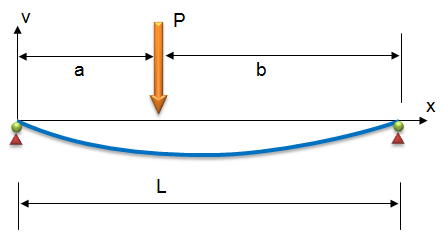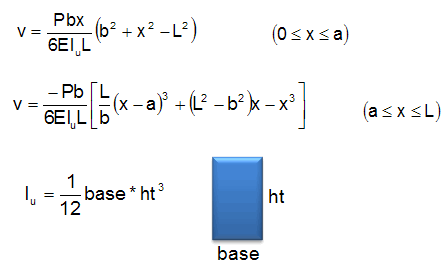# HW#7 - Simply Supported Beam

The following diagram represents a simply supported beam:The load (P) is offset from the left end of the beam by a distance (a).  The following equations can be used to compute the vertical deflection (v) at any point (x) along the length of the beam:Notice that the deflection equation is different depending on whether x is less than or greater than a.

a.  Write a spreadsheet that has the following inputs:

 Load (P): 5000 [lb] Modulus (E): 10000000 [lb/in^2] Length (L): 144 [in] Load Offset (a): 48 [in] Load Offset (b): [in] Base (base): 2 [in] Height (ht): 4 [in] Distance (x): 24 [in] Moment of Inertia (Iu): [lb/in^2] Deflection (v): [in]

(you will enter formulas for the values marked in blue)

Write the equations for the blue cells using the values in the yellow input cells. Note that the deflection will be computed as a negative value. For the deflection equation, you should enter both equations into a single cell with the two equations embedded in the IF function. (Hint: You may find it useful to write the equations into individual cells and then reference those cells within your IF statement.)

b. Use input validation (Data|Data Validation) to make sure the values entered for a and x are between zero and the value of L. (You should use the Between and Decimal options for this.)

c. Using the input values shown above, use the Goal Seek command (Data|What-If Analysis|Goal Seek) to compute the two x locations that result in a deflection of -2.0 inches.  Write your answer somewhere on your spreadsheet for the TA to see. (Hint: Goal seek will find the answer closest to the pre-existing x value so change x after finding the first solution.)

d. Using the input values shown above, use the Solver tool to find the x value resulting in the maximum deflection (v).  Note that you will actually be finding a minimum value since the deflection is negative.  When running the Solver, you will need to add some constraints so that x does not go less than zero or greater than L.  Write your answer (both the deflection and the corresponding x value) somewhere on your spreadsheet for the TA to see.

Remember that the Solver tool is located in the Data|Analysis tab. It is an Add-In, so it may be turned off by default in the copy of Excel you are using. If so, bring up the Help utility and look up the instructions for turning it on.